JAY PEAK

Face Chutes @ Jay Peak, VT: 239 vertical over 398 length = 30.98*.
133 vertical over 200 length = 33.62*.
70 vertical over 100 length = 34.99*.
40 vertical over 52 length = 37.57*.
Tuckerman's Chute @ Jay Peak, VT: 213 vertical over 324 length = 33.32*.
136 vertical over 200 length = 34.22*.
80 vertical over 115 length = 34.82*.
Green Beret @ Jay Peak, VT: 406 vertical over 775 length = 27.65*.
152 vertical over 202 length = 36.96*.
Timbuktu @ Jay Peak, VT: 571 vertical over 1303 length = 23.66*.
Upper Jet @ Jay Peak, VT: 602 vertical over 1366 length = 23.78*.
472 vertical over 1000 length = 25.27*.
240 vertical over 500 length = 25.64*.
110 vertical over 203 length = 28.45*.
Upper Can Am @ Jay Peak, VT: 718 vertical over 1563 length = 24.67*.
515 vertical over 1001 length = 27.23*.
290 vertical over 500 length = 30.11*.
123 vertical over 200 length = 31.59*.
Vertigo @ Jay Peak, VT: 637 vertical over 1456 length = 23.63*.
486 vertical over 1005 length = 25.81*.
270 vertical over 501 length = 28.32*.
116 vertical over 204 length = 29.62*.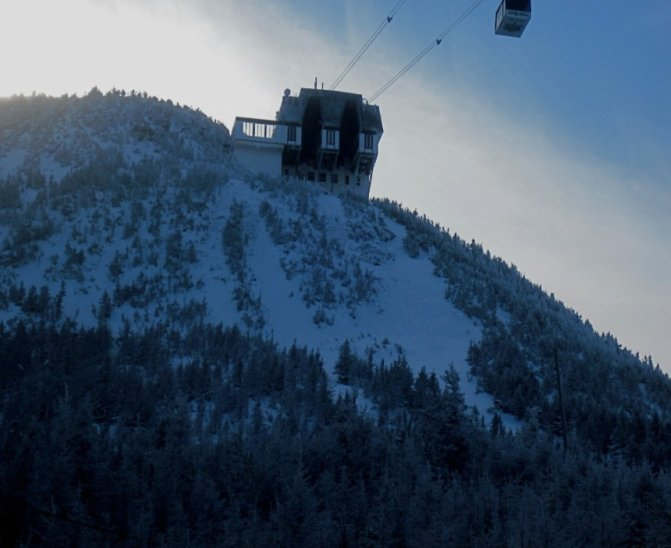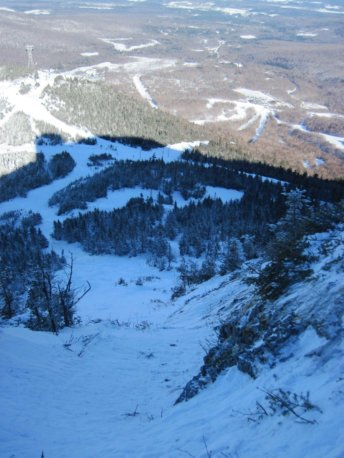Face Chutes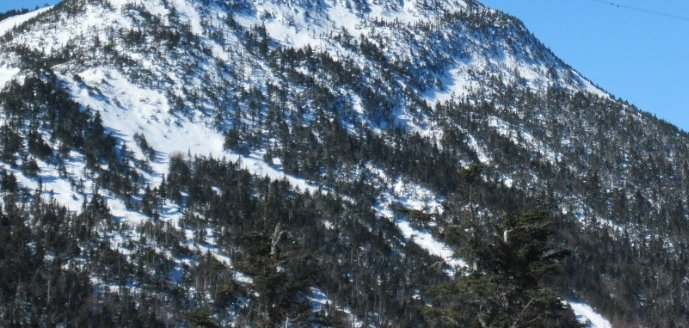Green Beret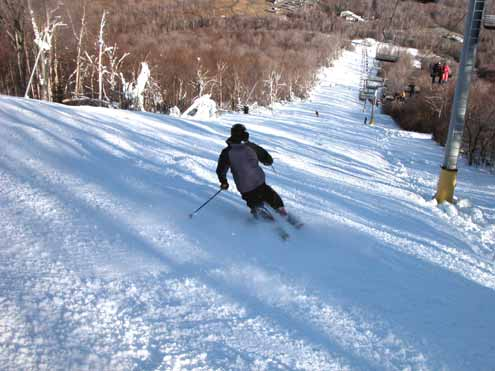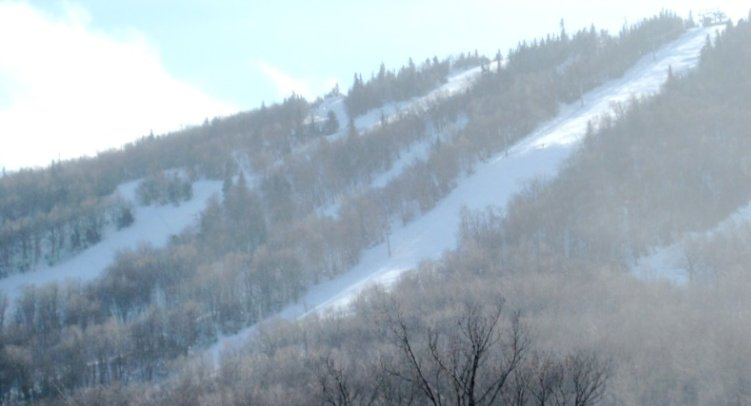The Jet (the wide one)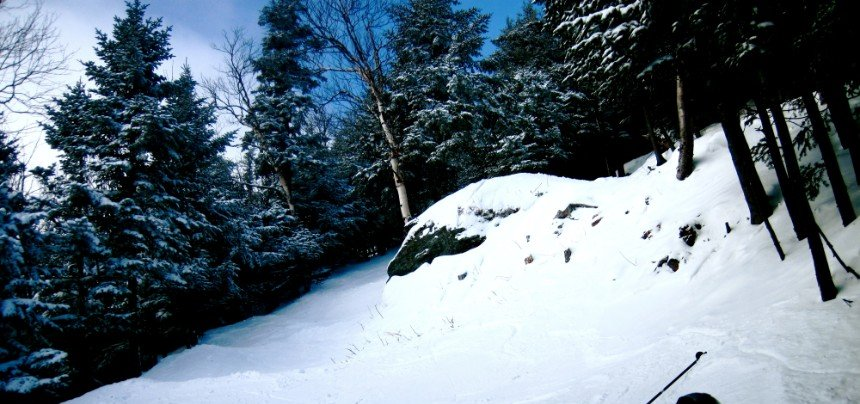Deliverance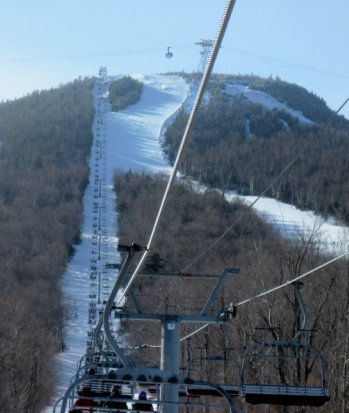Can Am is the wide trail. The trees to skier's left are Vertigo.

KILLINGTON

Outerlimits @ Killington, VT: 1002 vertical over 1892 length = 29.91*. Boasts to be the steepest and longest mogul run in New England.
626 vertical over 1064 length = 30.47*.
280 vertical over 432 length = 32.95*.
125 vertical over 154 length = 39.07*. This goes to prove that Outerlimits has some very steep sections.
376 vertical over 828 length = 24.42*. And this goes to prove that Outerlimits has some not-so-steep sections (numbers besides above numbers)
Devil's Fiddle @ Killington, VT: 998 vertical over 2170 length = 24.7*
190 vertical over 283 length = 33.88*. This is the headwall.
127 vertical over 158 length = 38.79*. This goes to prove that although Devil's Fiddle's overall is weak, its headwall is pretty darn steep.
808 vertical over 1887 length = 23.18*. And yet the headwall is so tiny compared to the rest of the run!
Lower Ovation @ Killington, VT: 468 vertical over 823 length = 29.62*
353 vertical over 553 length = 32.55*
146 vertical over 190 length = 37.54*.
113 vertical over 127 length = 41.66*.
95 vertical over 98 length = 44.11*. That's some pretty steep stuff.
Downdraft @ Killington, VT: 802 vertical over 1646 length = 25.98*.
273 vertical over 500 length = 28.63*.
182 vertical over 325 length = 29.25*.
129 vertical over 212 length = 31.32*.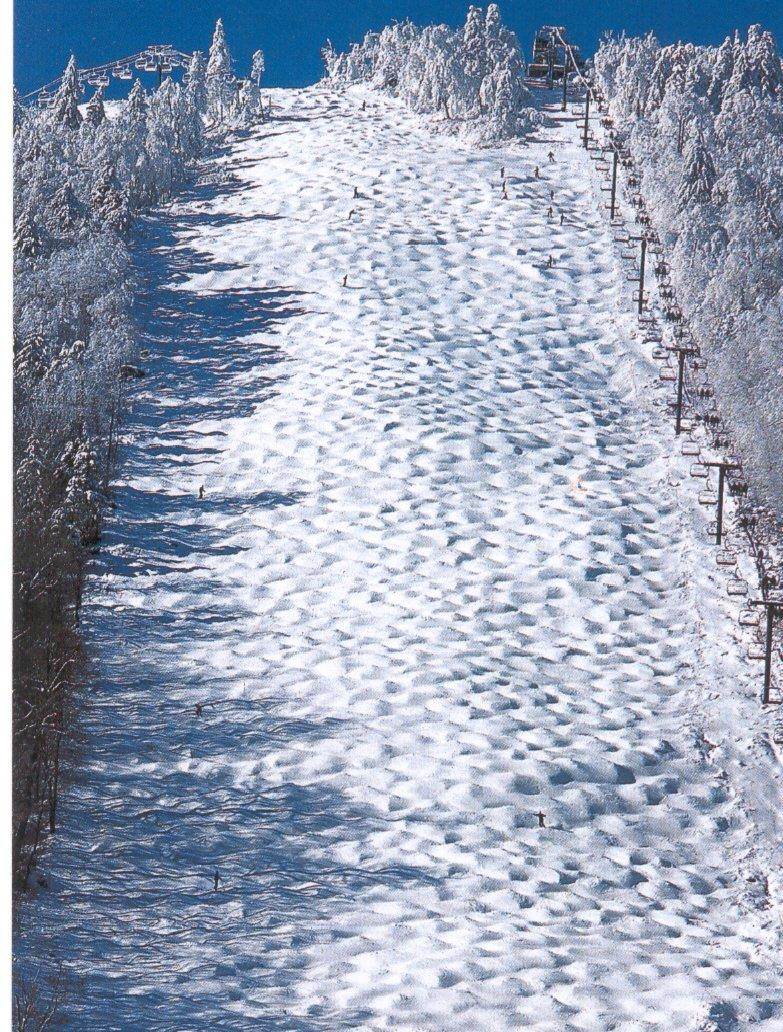Outer Limits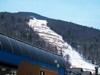Lower Ovation

Paradise @ Mad River Glen, VT: 698 vertical over 1208 length = 30.02*. Steepness means very little when it comes to Paradise.
382 vertical over 558 length = 34.4*.
150 vertical over 206 length = 36.06*.
119 vertical over 143 length = 39.77*.
Fall Line @ Mad River Glen, VT: 650 vertical over 1337 length = 25.93*
Chute @ Mad River Glen, VT: 577 vertical over 1258 length = 24.64*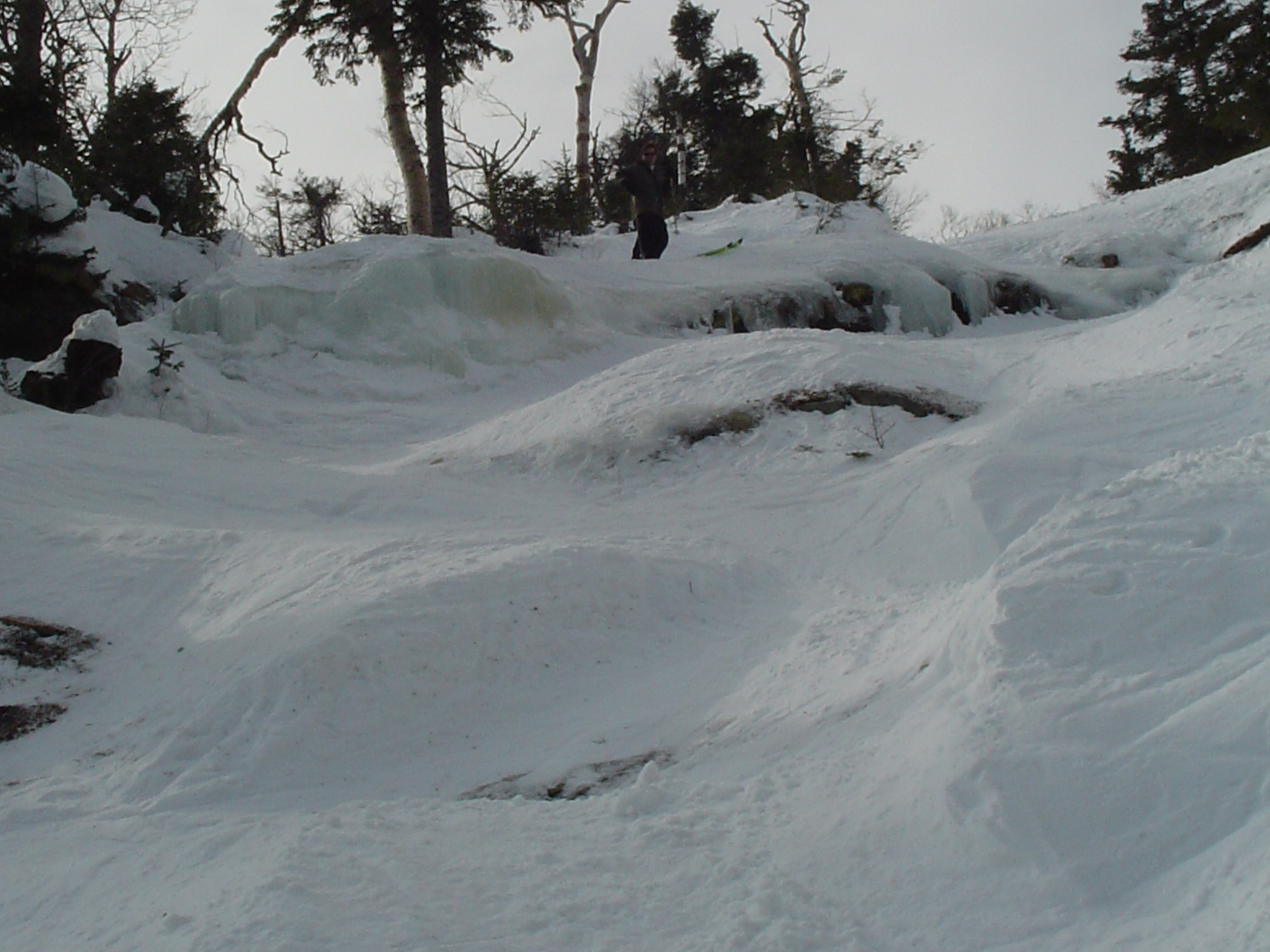Ripcord @ Mount Snow,VT: 269 vertical over 500 length = 28.28*. From top to the bottom of fall line, does not include flat top. Boasts 37 degree pitch.
141 vertical over 238 length = 30.64*.
61 vertical over 90 length = 34.13*. Closer look at the above numbers.
128 vertical over 262 length = 26.04*. This is the slope besides the above numbers.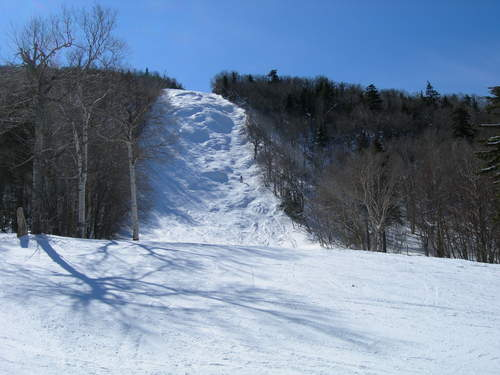Ripcord

SMUGGLERS NOTCH
Freefall @ Smugglers Notch, VT: 578 vertical over 946 length = 31.42*.
314 vertical over 456 length = 34.55*.
190 vertical over 231 length = 39.44*.
Black Hole @ Smugglers Notch, VT: 620 vertical over 1062 length = 30.28*.
341 vertical over 491 length = 32.6*. The top half (before the traverse to Robin's Run)
182 vertical over 253 length = 35.73*.
279 vertical over 571 length = 26.04*. The bottom half (after the traverse to Robin's Run). These are the numbers besides the numbers above.
Upper Liftline @ Smugglers Notch, VT: 1461 vertical over 3668 (almost 7/10 of a mile!) = 21.72*.
237 vertical over 294 length = 38.87*.
Robin's Run @ Smugglers Notch, VT: 320 vertical over 712 length = 24.2*.
279 vertical over 530 length = 27.76*.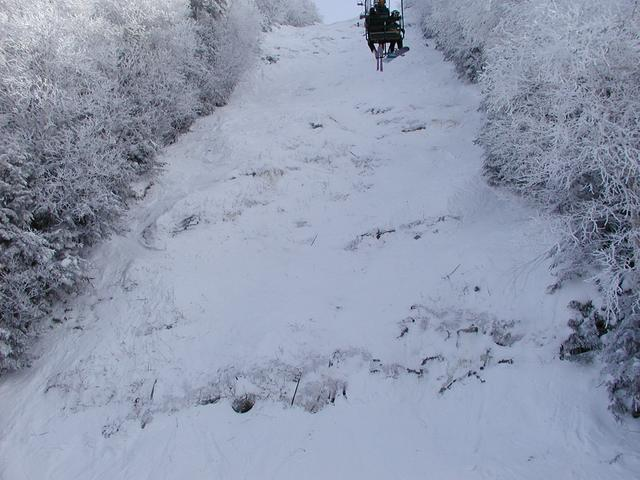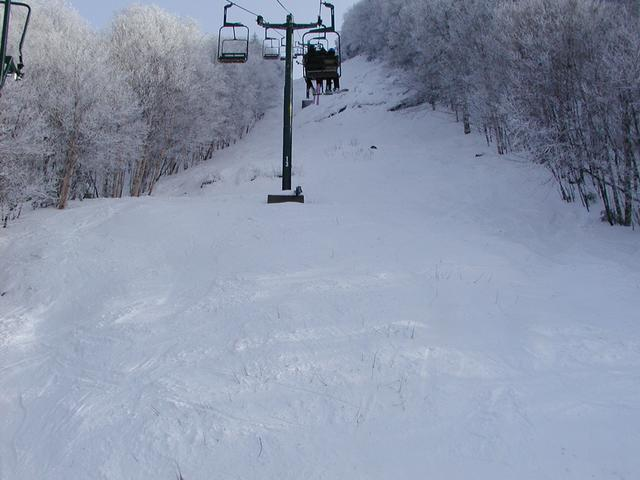Upper Liftline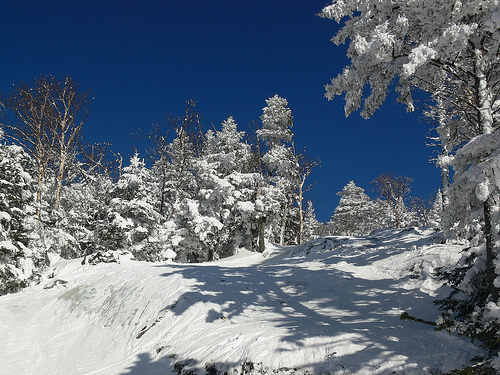Robin's Run

Goat @ Stowe, VT: 1180 vertical over 2441 length = 25.8*.

151 vertical over 195 length = 37.75*. Found on Upper Goat.
Starr @ Stowe, VT: 1068 vertical over 2193 length = 25.97*.
209 vertical over 284 length = 35.7*. Found on Upper Starr
National @ Stowe, VT: 1135 vertical over 2361 length = 25.67*.
188 vertical over 258 length = 36.08*. Found on Upper National.
Liftline @ Stowe, VT: 1363 vertical over 2974 length = 24.62*.
157 vertical over 240 length = 33.19*. Found on Liftline (middle portion).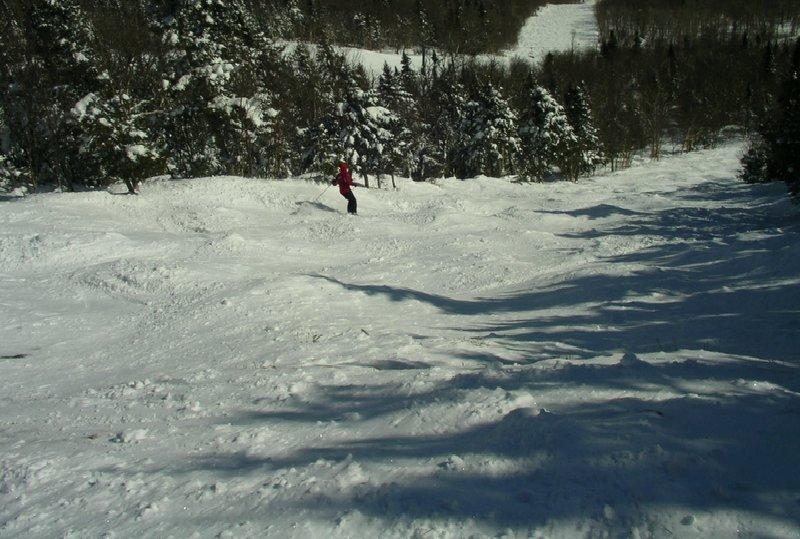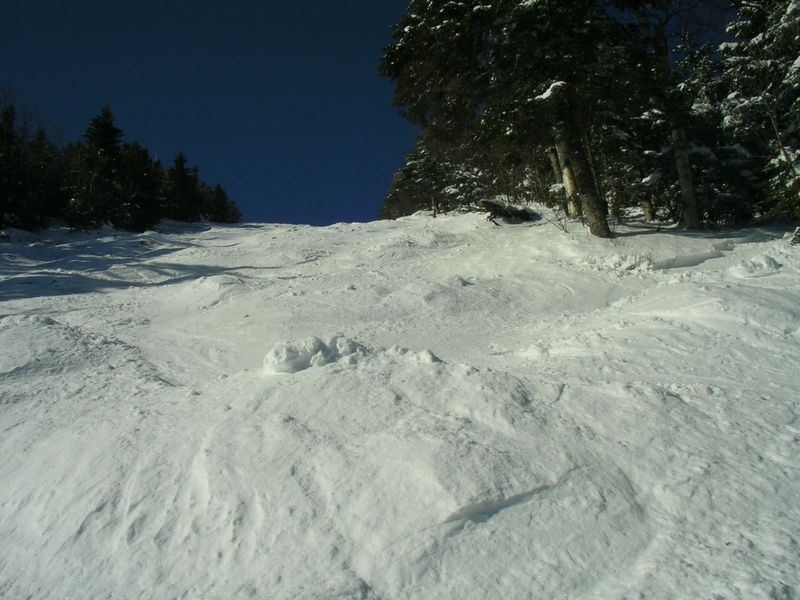Starr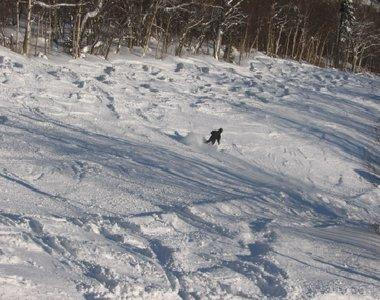Liftline

STRATTON
Beardown @ Stratton, VT: 660 vertical over 1690 length = 21.33*.
200 vertical over 429 length = 24.99*
World Cup @ Stratton: 270 vertical over 717 length = 20.63*.
111 vertical over 231 length = 25.67*.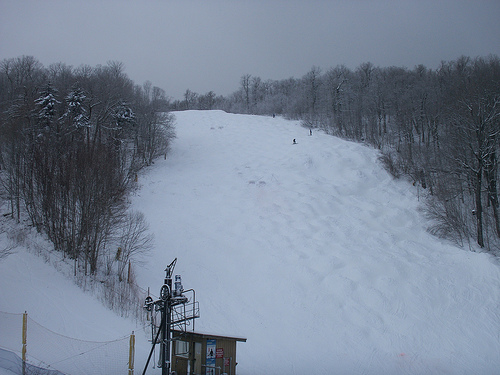Top of World Cup

Upper FIS @ Sugarbush, VT: 420 vertical over 741 length = 29.54*
174 vertical over 264 length = 33.39*
Black Diamond @ Sugarbush, VT: 473 vertical over 832 length = 29.62*
178 vertical over 264 length = 33.99*. As a liftline, it is much narrower than Upper FIS and slightly steeper and longer. They run side by side.
Rumble @ Sugarbush, VT: 735 vertical over 1723 length = 23.1*
224 vertical over 370 length = 31.19*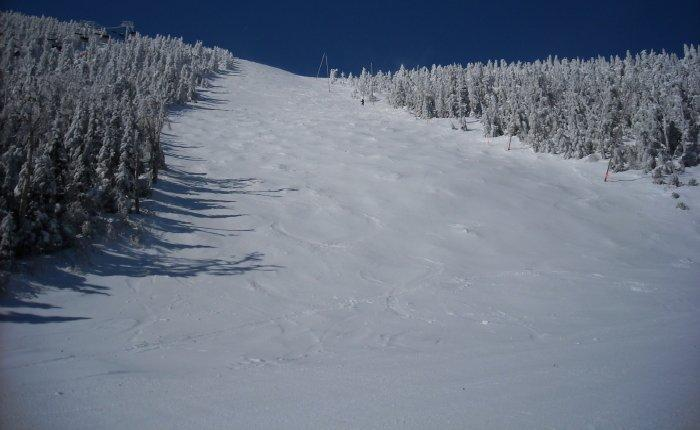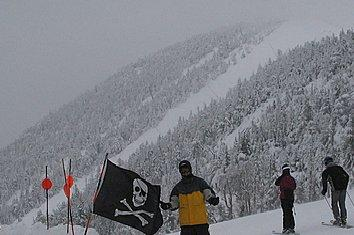Upper FIS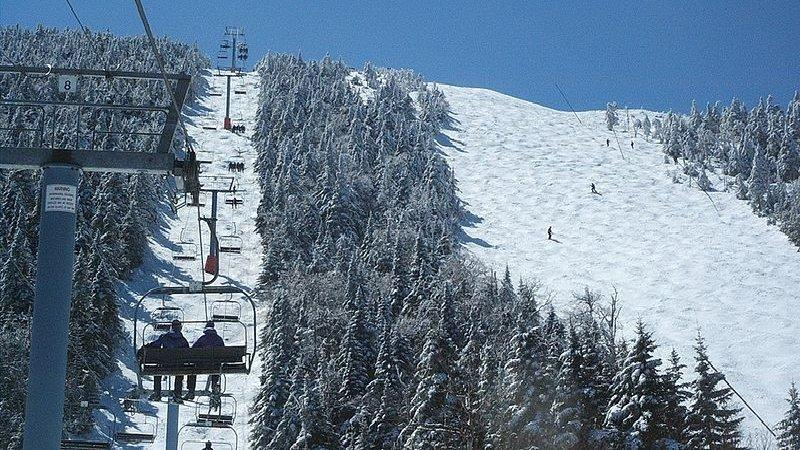Black Diamond (left, it is the narrow liftline) and Upper FIS (wide run on the right)

SUICIDE SIX
Back Scratcher @ Suicide Six, VT: 369 vertical over 995 length = 20.35*.
126 vertical over 228 length = 28.93*.
67 vertical over 100 length = 33.82*.
The Face @ Suicide Six, VT: 513 vertical over 1442 length = 19.58*.
303 vertical over 753 length = 21.92*.
126 vertical over 221 length = 29.69*.
Make a Free Website with Yola.